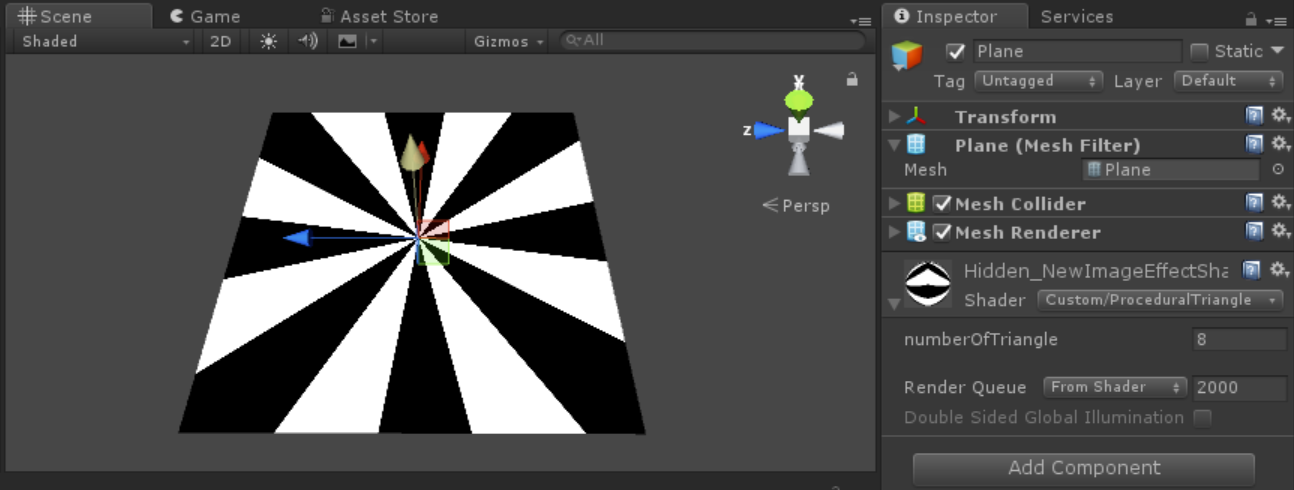# Draw triangles around screen

I am trying to draw triangles around the screen something like this:This is my script which can draw 6 triangles but I am not sure how to expand it to draw more like 8 or 10 triangles. Any help is appreciated

using UnityEngine;
using System.Collections;

public class BackgroundScript : MonoBehaviour
{
[SerializeField]
private Material darkMaterial;
[SerializeField]
private Material lightMaterial;

[SerializeField]
private float triangleDistance = 50;

private GameObject[] triangles;

void Start()
{
CreateBackground();
}

private void CreateBackground()
{
float w = triangleDistance * Mathf.Tan(30 * (Mathf.PI / 180));

triangles = new GameObject;

for (int i = 0; i < 6; i++)
{
GameObject backgroundTriangle = new GameObject();
backgroundTriangle.name = "triangle " + i;
triangles[i] = backgroundTriangle;

Mesh mesh = new Mesh();
mesh.vertices = new Vector3[] { new Vector3(0, 0, 0), new Vector3(triangleDistance, 0, w), new Vector3(triangleDistance, 0, -w) };
mesh.normals = new Vector3[] { new Vector3(0, 1, 0), new Vector3(0, 1, 0), new Vector3(0, 1, 0) };
mesh.triangles = new int[] { 0, 1, 2 };
mf.mesh = mesh;

mr.material = i % 2 == 0 ? darkMaterial : lightMaterial;

backgroundTriangle.transform.Rotate(new Vector3(0, i * 60 + 30, 0));
backgroundTriangle.transform.SetParent(this.transform);
}
}
}

• There is clearly a 6 twice in your code...Also you don't need to create a new mesh for every triangle. You could just push all the triangles into one mesh for each material. – Sidar Mar 17 '18 at 14:14
• @Sidar Even if you change the 6 to 8 or 10 nothing really happens. I believe that is not the issue – Casanova Mar 17 '18 at 17:32
• Oh but it is, you should also consider how the angles are calculated. – Sidar Mar 17 '18 at 17:33
• @Sidar Yeah definitely angles – Casanova Mar 17 '18 at 20:10

eight triangle:using UnityEngine;
using System.Collections;

public class BackgroundScript : MonoBehaviour
{
[SerializeField]
private Material darkMaterial;
[SerializeField]
private Material lightMaterial;

[SerializeField]
private float triangleDistance = 50;

private GameObject[] triangles;

void Start()
{
CreateBackground();
}

private void CreateBackground()
{
float w = triangleDistance * Mathf.Tan(22 * (Mathf.PI / 180));

triangles = new GameObject;

for (int i = 0; i < 8; i++)
{
GameObject backgroundTriangle = new GameObject();
backgroundTriangle.name = "triangle " + i;
triangles[i] = backgroundTriangle;

Mesh mesh = new Mesh();
mesh.vertices = new Vector3[] { new Vector3(0, 0, 0), new Vector3(triangleDistance, 0, w), new Vector3(triangleDistance, 0, -w) };
mesh.normals = new Vector3[] { new Vector3(0, 1, 0), new Vector3(0, 1, 0), new Vector3(0, 1, 0) };
mesh.triangles = new int[] { 0, 1, 2 };
mf.mesh = mesh;

mr.material = i % 2 == 0 ? darkMaterial : lightMaterial;

backgroundTriangle.transform.Rotate(new Vector3(0, i * 45 + 30, 0));
backgroundTriangle.transform.SetParent(this.transform);
}
}
}


also you can create this shape by shader.

The Book of Shaders Shapes Tutorial

To create a new shader, either choose Assets > Create > Shader > Unlit Shader from the main menu, or duplicate an existing shader and work from that. The new shader can be edited by double-clicking it in the Project View.then copy below shader to it:

 Shader "Custom/ProceduralTriangle"
{
Properties
{
_numberOfTriangle("numberOfTriangle",Int) = 8
}
{

Pass
{
CGPROGRAM
#pragma vertex vert
#pragma fragment frag

#include "UnityCG.cginc"

struct appdata
{
float4 vertex : POSITION;
float2 uv : TEXCOORD0;
};

struct v2f
{
float2 uv : TEXCOORD0;
float4 vertex : SV_POSITION;
};

v2f vert (appdata v)
{
v2f o;
o.vertex = UnityObjectToClipPos(v.vertex);
o.uv = v.uv;
return o;
}

int _numberOfTriangle;

fixed4 frag (v2f i) : SV_Target
{
float2 st = i.uv;
float3 color = float3(0,0,0);

float2 pos = float2(0.5,0.5)-st;

float r = step(length(pos),0.);
float a = atan2(pos.x,pos.y);

float f = cos(a*_numberOfTriangle+_Time.y);
float c = smoothstep(f,f+0.02,r);
color = float3(c,c,c);

return float4(color, 1.0);
}
ENDCG
}
}
}


then create a material and use this shader.this is simple fragment shader if you want to create geometry you can use geometry shader.I will update my question and explain this.

• I made an empty gameobject. Add this to it but nothing happens – user113599 Mar 17 '18 at 21:25
• @user113599 shader don't work in empty object try it again by using Plane object.if you like to use this shader in gameObject first you should create a shader and attach it to material then use it. – Seyed Morteza Kamali Mar 18 '18 at 3:11
• Hmm would be great if you can add a geometry shader as well – Casanova Mar 18 '18 at 4:25